Courses

# RD Sharma Solutions - Ex-23.3, Data Handling II Central Values, Class 7, Math Class 7 Notes | EduRev

## Class 7: RD Sharma Solutions - Ex-23.3, Data Handling II Central Values, Class 7, Math Class 7 Notes | EduRev

The document RD Sharma Solutions - Ex-23.3, Data Handling II Central Values, Class 7, Math Class 7 Notes | EduRev is a part of the Class 7 Course RD Sharma Solutions for Class 7 Mathematics.
All you need of Class 7 at this link: Class 7

#### Question 1:

Find the median of the following data (1-8)
83, 37, 70, 29, 45, 63, 41, 70, 34, 54

Arranging the data in ascending order, we have:

29, 34, 37, 41, 45, 54, 63, 70, 70, 83

Here, the number of observations, n = 10 (Even).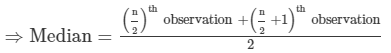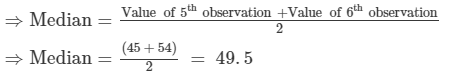Hence, the median of the given data is 49.5.

#### Question 2:

Find the median of the following data (1-8)
133, 73, 89, 108, 94, 104, 94, 85, 100, 120

Arranging the data in ascending order, we have:

73, 85, 89, 94, 94, 100, 104, 108, 120, 133.

Here, the number of observations n = 10 (Even).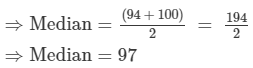Hence, the median of the given data is 97.

#### Question 3:

Find the median of the following data (1-8)
31, 38, 27, 28, 36, 25, 35, 40

Arranging the data in ascending order, we have:

25,27, 28, 31, 35, 36, 38, 40

Here, the number of observations n = 8 (Even).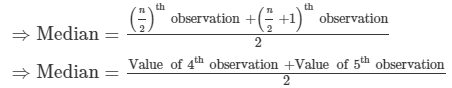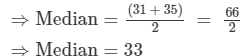Hence, the median of the given data is 33.

#### Question 4:

Find the median of the following data (1-8)
15, 6, 16, 8, 22, 21, 9, 18, 25

Arranging the data in ascending order, we have:

6, 8, 9, 15,16,18, 21, 22, 25

Here, the number of observations n = 9 (Odd).

⇒Median=Value of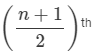observati5on i.e , value of the 5th observation  = 16

Hence, the median of the given data is 16

#### Question 5:

Find the median of the following data (1-8)
41, 43, 127, 99, 71, 92, 71, 58, 57

Arranging the given data in ascending order, we have:

41, 43, 57, 58, 71,71, 92, 99, 127
Here, n = 9, which is odd.

∴ Median = Value of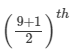observation, i.e., the 5th observation = 71.

#### Question 6:

Find the median of the following data (1-8)
25, 34, 31, 23, 22, 26, 35, 29, 20, 32

Arranging the given data in ascending order, we have:

20, 22, 23, 25, 26, 29, 31, 32, 34, 35

Here, n = 10, which is even.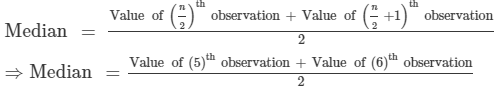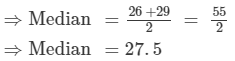Hence, the median is 27.5 for the given data.

#### Question 7:

Find the median of the following data (1-8)
12, 17, 3, 14, 5, 8, 7, 15

Arranging the given data in ascending order, we have:

3,5,7,8,12,14,15,17

Here, n = 8, which is even.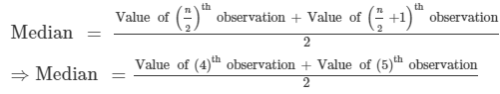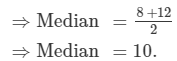Hence, the median of the given data is 10.

#### Question 8:

Find the median of the following data (1-8)
92, 35, 67, 85, 72, 81, 56, 51, 42, 69

Arranging the given data in ascending order, we have:

35, 42, 51, 56, 67, 69, 72, 81, 85, 92

Here, n = 10, which is even.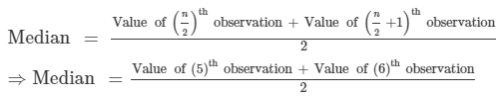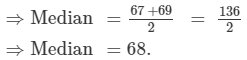Hence, the median of the given data is 68.

#### Question 9:

Numbers 50, 42, 35, 2x + 10, 2x − 8, 12, 11, 8, 6 are written in descending order and their median is 25, find x.

Here, the number of observations n is 9. Since n is odd , the median is the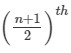observation, i.e. the 5th  observation.
As the numbers are arranged in the descending order, we therefore observe from the last.
Median =  5th  observation.
⇒ 25 = 2x -8
⇒ 2x = 25 +8
⇒ 2x = 33
x = 33/2
x = 16.5
Hence, x = 16.5.

#### Question 10:

Find the median of the following observations : 46, 64, 87, 41, 58, 77, 35, 90, 55, 92, 33. If 92 is replaced by 99 and 41 by 43 in the above data, find the new median?

Arranging the given data in ascending order, we have:

33, 35, 41, 46, 55, 58, 64, 77, 87, 90, 92

Here, the number of observations n is 11 (odd).

Since the number of observations is odd, therefore,

Median = Value of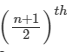observation = Value of the 6th observation = 58.
Hence, median = 58.
If 92 is replaced by 99 and 41 by 43, then the new observations arranged in ascending order are:
33, 35, 43, 46, 55, 58, 64, 77, 87, 90, 99.
∴ New median =  Value of the 6th observation = 58.

#### Question 11:

Find the median of the following data : 41, 43, 127, 99, 61, 92, 71, 58, 57, If 58 is replaced by 85, what will be the new median?

Arranging the given data in ascending order, we have:

41, 43, 57, 58, 61, 71, 92, 99,127

Here, the number of observations, n, is 9(odd).

∴ Median = Value ofobservation = Value of the 5th observation = 61.
Hence, the median = 61.
If 58 is replaced by 85 , then the new observations arranged in ascending order are:
41, 43, 57,  61, 71, 85, 92, 99,12 .
∴ New median =  Value of the 5th observation = 71.

#### Question 12:

The weights (in kg) of 15 students are : 31, 35, 27, 29, 32, 43, 37, 41, 34, 28, 36, 44, 45, 42, 30. Find the median. If the weight 44 kg is replaced by 46 kg and 27 kg by 25 kg, find the new median.

Arranging the given data in ascending order, we have:

27, 28, 29, 30, 31, 32, 34, 35, 36, 37, 41, 42, 43, 44, 45.

Here, the number of observations n is 15(odd).

Since the number of observations is odd, therefore,

Median = Value ofobservation = Value of the 8th observation = 35.
Hence, median = 35 kg.

If 44 kg is replaced by 46 kg and 27 kg by 25 kg , then the new observations arranged in ascending order are:
25, 28, 29, 30, 31, 32, 34, 35, 36, 37, 41, 42, 43, 45, 46.
∴ New median =  Value of the 8th observation = 35 kg.

#### Question 13:

The following observations have been arranged in ascending order. If the median of the data is 63, find the value of x :
29, 32, 48, 50, xx + 2, 72, 78, 84, 95

Here, the number of observations n is 10. Since n is even,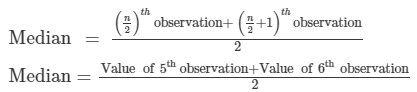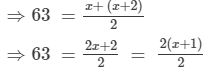⇒63 = x+1

⇒x = 63−1 = 62.
Hence, x = 62.

The document RD Sharma Solutions - Ex-23.3, Data Handling II Central Values, Class 7, Math Class 7 Notes | EduRev is a part of the Class 7 Course RD Sharma Solutions for Class 7 Mathematics.
All you need of Class 7 at this link: Class 7Use Code STAYHOME200 and get INR 200 additional OFF Use Coupon Code
All Tests, Videos & Notes of Class 7: Class 7

### Up next### Top Courses for Class 7## RD Sharma Solutions for Class 7 Mathematics

97 docs

### Up next### Top Courses for Class 7Track your progress, build streaks, highlight & save important lessons and more!

,

,

,

,

,

,

,

,

,

,

,

,

,

,

,

,

,

,

,

,

,

,

,

,

,

,

,

,

,

,

;